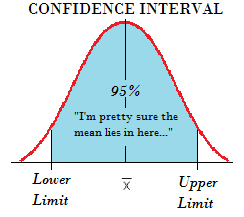# Confidence Limits: Definition

Share onConfidence limits are a pair of numbers used to describe an estimate or other characteristic of a population. They are the upper and lower boundaries of confidence intervals . Anywhere you calculate a confidence interval (e.g., effect sizes, noncentrality parameters, risk ratios), you will have associated confidence limits. It represents limits between which you expect to find a particular measurement or statistic.

More precisely, a confidence limit is a range of values, calculated around a sample statistic, that contains the true parameter with given probability α for repeated sampling. A 5% alpha level is commonly used, which is associated with a 95% confidence level. If you were to repeat your experiment over and over again, 95% of the time the true population parameter would lie between the specified lower limit and upper limit. For example, if your confidence interval for a mean (μ) is 5 < μ < 6, your confidence limits are 5 and 6. If you were to repeat this experiment, 95% of the time you would expect to find the true population parameter within these limits. There is a 5% chance that the results would not contain the true value.

## 95% Confidence Limits Example

Example question: Find the confidence limits for a 95% confidence interval for a mean of 67.45 and a standard deviation of 2.93.

Solution: The confidence limits associated with a 95% confidence interval can be found with the formula
x̄ ± 1.96σ / √(n).
Plugging the statistics given in the question into the formula gives :

67 ± 1.96(2.93/√(100), or 67.45 ± 0.57.

Our confidence interval can be written as 66.88 < μ < 68.02. The confidence limits — the upper and lower end of the confidence interval — are 66.88 and 68.02. If we were to repeat our experiment over and over, 95% of the time our samples will fall between 66.88 and 68.02.

## References

 William, R. Confidence Intervals. Retrieved December 6, 2021 from: https://www3.nd.edu/~rwilliam/stats1/x23.pdf
 Estimation theory.

CITE THIS AS:
Stephanie Glen. "Confidence Limits: Definition" From StatisticsHowTo.com: Elementary Statistics for the rest of us! https://www.statisticshowto.com/confidence-limits-definition/
---------------------------------------------------------------------------Need help with a homework or test question? With Chegg Study, you can get step-by-step solutions to your questions from an expert in the field. Your first 30 minutes with a Chegg tutor is free!